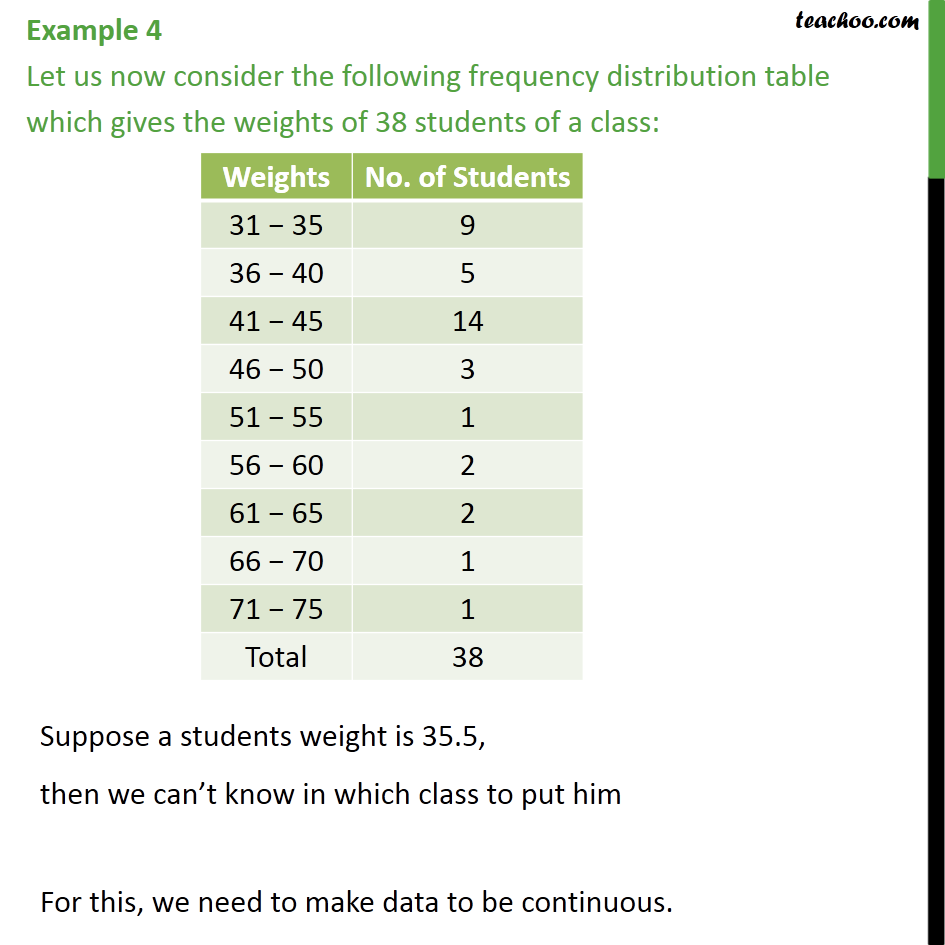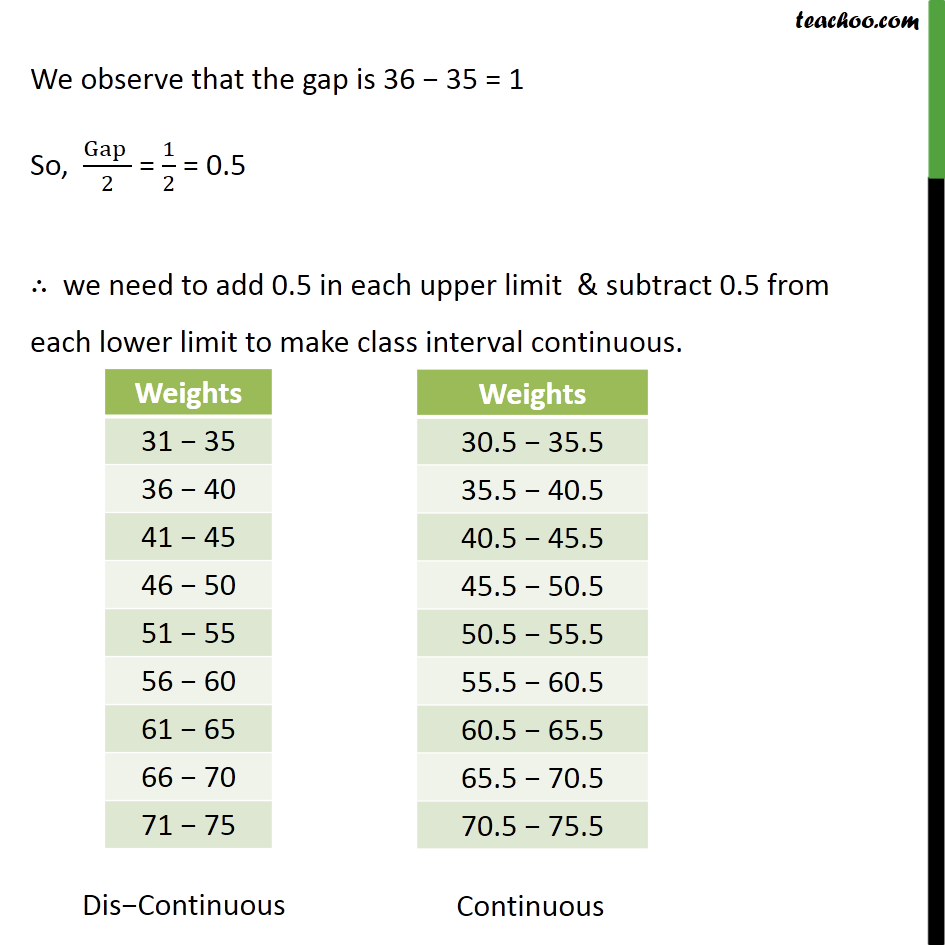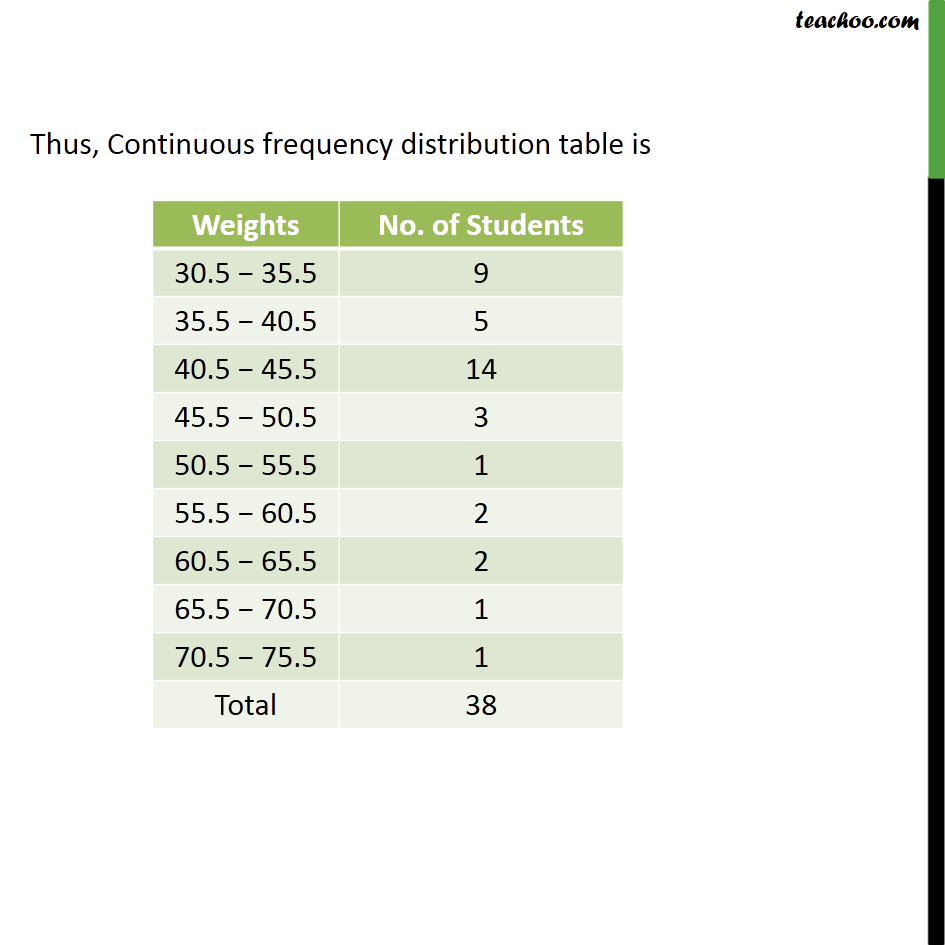Examples

Chapter 12 Class 9 Statistics
Serial order wiseLearn in your speed, with individual attention - Teachoo Maths 1-on-1 Class

### Transcript

Question 4 Let us now consider the following frequency distribution table which gives the weights of 38 students of a class: Suppose a students weight is 35.5, then we can t know in which class to put him For this, we need to make data to be continuous. We observe that the gap is 36 35 = 1 So, (Gap )/2 = 1/2 = 0.5 we need to add 0.5 in each upper limit & subtract 0.5 from each lower limit to make class interval continuous. Thus, Continuous frequency distribution table is